Published: 30 June 2014

# Numerical calculation and finite element calculation on impeller of stainless steel multistage centrifugal pump

Chuan Wang1
Weidong Shi2
Qiaorui Si3
Ling Zhou4
1, 2, 3, 4National Research Center of Pumps, Jiangsu University, Zhenjiang, 212013, China
4Department of Mechanical Engineering and Material Science Washington University in St. Louis, St. Louis, Missouri, USA
Corresponding Authors:
Chuan Wang, Ling Zhou
Views 375

#### Abstract

In order to save energy and materials, some mechanical structures are very thin. Aiming to study the influence of impeller blade thickness on the performance of stainless steel multistage centrifugal pump, the whole flow field of stainless steel multistage centrifugal pump with different blade thickness were calculated based on ANSYS Fluent. The relationship between the impeller blade thickness and the overall performance of the pump was analyzed. To further study the reliability of the impeller structure for stainless steel multistage centrifugal pump, based on the ANSYS Workbench, the final stage impeller of the pump with different blade thickness were calculated by using the finite element method. Results indicate that with the increase of blade thickness, the maximum stress and deformation of the impeller gradually decreased, while the stability of the impeller structure increased.

## 1. Introduction

It is becoming a world consensus to implement energy-saving and emission reduction because of global energy crisis and soaring atmospheric temperature . Some researchers go on developing new energy sources , while others prefer to save energy in existing machinery. As a significant device for energy conversion and fluid transportation, pump is widely used in national economic fields. It is not exaggerated to say that there is an operating pump wherever fluid is flowing [3-5].

Stainless steel multistage centrifugal pump is specially equipped for groundwater extraction in rural areas, factories, mines, water supply companies, geothermal development, and oil fields . It is mainly produced through some advanced procedures including laying-off, stamping, stretching, bulging, shaping, welding, polishing, and etc. [6-8]. Impeller is the key component of stainless steel multistage centrifugal pump, whose blade thickness determined by the steel sheet can reach 0.5 mm. The blade thickness is one of the main parameters in pump design, which makes the traditional design method of casting pump is not fully applicable to stainless steel pump. Although thin blade causes energy-saving effects, it may also influence the reliability of impeller structure. Hence, it has a great realistic significance to study the stress and deformation on impeller with different blade thicknesses.

In the past, some papers studied on the blade thickness in the rotational machinery. C. Sarraf  did the experimental study of blade thickness effects on the overall and local performances of a controlled vortex designed axial-flow fan, and the results show the efficiency of the thick blades fan is lower than the efficiency of the fan with thin blades but remains high on a wider flow-rate range, and the mean velocity fields downstream of the rotors are very similar at nominal points with less centrifugation for the thick blades fan. D. Chapple  did the effect of impeller and tank geometry on power number for a pitched blade turbine, and the results show that the power number is independent of blade thickness, but dependent on the impeller to tank diameter ratio. C. Galletti  did the effect of shaft eccentricity and impeller blade thickness on the vortices features in an unbaffled vessel, and the results show the characteristic frequency of flow instabilities was found to increase with reducing eccentricity or impeller blade thickness. However, few papers studied on the blade thickness in the centrifugal pump.

The strength calculation on impeller of centrifugal pumps is currently based on empirical formulas, but the formulas have simplified greatly the load on impeller. As a result, there are fairly large errors between the calculated value and actual value. With the continuous improvement of computer performance, numerical calculation develops rapidly [12, 13], and Computational Fluid Dynamics has been widely used in the pump design [14-16]. Flow field calculation and strength calculation can be combined through fluid structure interaction (FSI), which makes the results close to actual value. So far most of contemporary studies on FSI of rotating machinery only focus on single stage centrifugal pump [17, 18].

With finite volume method, this study implements flow field calculation on impeller with five different blade thicknesses by ANSYS Fluent and then analyzes the changing law of pump's performance. Based on ANSYS Workbench, this paper studies the final stage impeller of stainless steel multistage pump with FSI and implements finite element calculation on impeller. As a reasonable value of blade thickness, 1.5 mm is selected at last.

## 2. Solving theory

### 2.1. Theory on flow field calculation

According to Bernoulli equation, pump experimental head is:

1
$H=\frac{{p}_{d}-{p}_{s}}{\rho g}+\frac{{v}_{d}^{2}-{v}_{s}^{2}}{2g}+\left({z}_{d}-{z}_{s}\right),$

where ${p}_{d}$ and ${p}_{s}$ are fluid static pressure on outlet and inlet section, ${v}_{d}$ and ${v}_{s}$ are fluid velocity on outlet and inlet section, ${z}_{d}$ and ${z}_{s}$ are the distances to base level from outlet and inlet section. The efficiency of pump is the ratio of effective power to shaft power, and is represented by:

2
$\eta =\frac{{P}_{\xi }}{{P}_{t}}=\frac{\rho gQH}{M\omega },$

where ${P}_{\xi }$ is the effective power, ${P}_{t}$ is the shaft power, $Q$is volume flow, $M$ is the sum torque on shaft, and $\omega$ is angular velocity of impeller. After applying the results of flow field calculation, the pump performance curve is obtained.

### 2.2. Theory on fluid structure interaction

Fluid solid interaction (FSI) mechanics is the interdisciplinary branch of fluid mechanics science and solid mechanics science. It mainly studies the deformation and movement of solids in the flow field, as well as the impact of this behavior to the flow field. In order to check the impeller strength and predict the exact location of maximum stress on impeller directly, flow field calculation and finite element calculation can be combined together by using FSI.

The solving of FSI problems is comprised of two-way coupling and unilateral coupling. The former one means all unknown quantities in both fluid field and solid field are solved at the same time step according to common coupling equations, while the latter is a kind partition iterative solving that means the data is unidirectional when transferring on coupling interface. For inner flow field, the solid deformation influence may be neglected because the deformation of impeller is very small. Thus, unidirectional FSI method is used in this paper and the flow table is shown in Fig. 1.

Fig. 1Computational flow table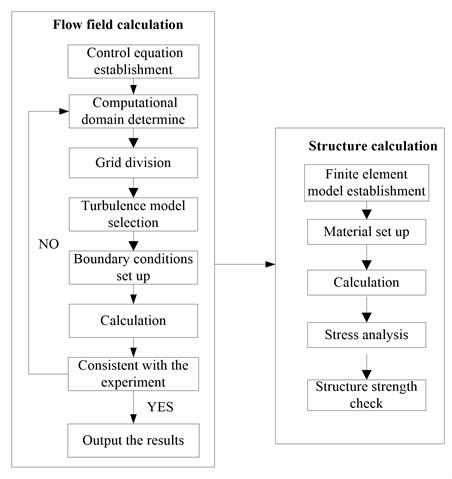## 3. Modeling

### 3.1. Hydraulic design of model pump

The stainless steel multistage centrifugal pump of 100XQJ8 is selected in the studies. Its design parameters are listed as below: rated flow $Q=$8 m3/h, single stage head $H=$4.5 m, rotational speed $n=$2850 r/min, maximum power $P=$3 kW, and the total head $H=$72 m. In view of the structural condition that the outer diameter of multistage well pump is limited by well diameter, Lu Weigang  studied a design method of well pump, the impeller maximal diameter design method, which expands the front shroud of impeller to the edge of pump wall and makes the impeller diameter achieve maximum value while well diameter is invariable.

Impeller with five different blade thicknesses (${\delta }_{1}=$0.5 mm, ${\delta }_{2}=$1.0 mm, ${\delta }_{3}=$1.5 mm, ${\delta }_{4}=$2.0 mm, and ${\delta }_{5}=$2.5 mm) are designed as research objects. The geometric parameters are shown in Table 1.

Table 1Geometric parameters of impeller

 Geometric parameter Value Blade number $Z$ 9 Blade inlet angle ${\beta }_{1}$ (°) 35 Blade outlet angle ${\beta }_{2}$ (°) 23.2 Blade wrap angle $\phi$ (°) 120 Front cover plate diameter ${D}_{2max}$ (mm) 79.5 Inlet diameter of impeller ${D}_{j}$ (mm) 39.4 Width of the blade outlet ${b}_{2}$ (mm) 5 Slope of impeller outlet $\theta$ (°) 29.4 Shaft diameter $d$ (mm) 12.5 External diameter of shaft sleeve ${d}_{h}$ (mm) 17

There is an impeller model displayed in Fig. 2(a), whose blade thickness is 1.5 mm. The impeller is in coordination with reverse diffuser, which can shorten the axial length of well pump to the minimum. The main parameters of reverse diffuser are listed as below: the number of blade $Z=$8, inlet width ${B}_{3}=$6.2 mm, inlet diameter ${D}_{5}=$75 mm, and outlet diameter ${D}_{6}=$37.5 mm. Reverse diffuser is shown in Fig. 2(b).

Fig. 2Three-dimensional diagram of impeller and reverse diffuser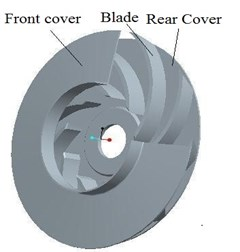a) Impeller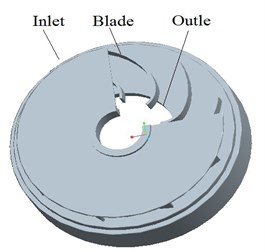b) Reverse diffuser

### 3.2.1. Computational domain

Stainless steel stamping centrifugal pump is one of multi-stage pumps. Compared with single-stage pump, the structure of multi-stage pump is more complex and its flow characteristics are different. Therefore, it’s particularly important to select appropriate series when making numerical simulation for multi-stage pump. I have ever done relevant research. Four different series in a numerical simulation with the blade thickness of 1.5 mm are selected and the efficiency and head at all series are shown in Table 3 and Table 4. As can be seen from Table 3 and Table 4, the efficiency and head of the first-stage in different stages are almost the same. When the series is $n\ge$2, there is little difference between the second stage and the following, but the difference is big compared with the first stage. It is also consistent with the fact that the flow in the first impeller inlet of multi-stage pump is usually irrotational while rotational in the other impeller inlet. Taking into account that increase of stage will bring about more cell number, thereby demand computer with higher performance, so the two-stage model is selected and the efficiency as well as head of the second stage are taken as performance prediction value. In the numerical calculation of the pump, the water part in the pump is meshed by many small cells. The pump water model is consist of inlet section (water), two impellers (water), two chambers (water), two reverse diffusers (water), outlet section (water), which is shown in Fig. 3.

Table 2Efficiency comparisons of different stages

 Series ($n$) 1 2 3 4 The first-stage efficiency (%) 59.51 59.93 59.79 59.86 The second-stage efficiency (%) 62.50 62.53 62.53 The third-stage efficiency (%) 62.59 62.58 The fourth-stage efficiency (%) 62.61 The total efficiency (%) 56.57 59.55 60.12 60.14

Table 3Head comparisons of different stages

 Series ($n$) 1 2 3 4 The first-stage head (m) 4.745 4.762 4.758 4.755 The second-stage head (m) 4.611 4.605 4.612 The third-stage head (m) 4.608 4.621 The fourth-stage head (m) 4.628 The average head (m) 4.511 4.588 4.595 4.597

Fig. 3Pump water model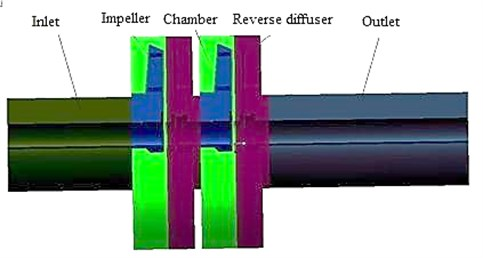### 3.2.2. Model mesh

In order to verify the quantity of cell affecting the computational accuracy, the experiment of cell independence is done. In theory, with the increase of the cell number, the solution caused by the cell will be gradually reduced until it disappears. But the more the cell number, the slower the computing speed, thus considering the configuration of the computer and the computing time, cell number can not be too much. For the above hydraulic model with the blade thickness of 1.5 mm, five different cell sizes of numerical calculation are selected; the results are shown in Table 4. As can be seen from Table 4, when the cell size is large, the efficiency and single head at rated point ($Q=$8 m3/h) are relatively small. If the cell size is less than 1.2 mm, with the decrease of cell size, the efficiency and single head almost remain invariable. Taking into account the coordination of computing time and accuracy, 1.2 mm of cell size meets the requirements, so the cell size in this paper is 1.2 mm. Also the detailed information of cell distribution is given as shown in Table 5. In order to verify the quality of the model mesh, the mesh of impeller and reverse diffuser are shown in Table 5.

Table 4Mesh independent analysis

 Parameters Cell size (mm) 1.0 1.2 1.4 1.6 1.8 Cell number 1912342 1176683 802300 563413 418491 Efficiency $\eta$ (%) 62.49 62.50 62.53 61.97 61.72 Single head $H$ (m) 4.612 4.611 4.605 4.551 4.483

Table 5Detailed information of grid distribution

 Component Grid number Inlet section 139083 First impeller 96007 First chamber 140078 First reverse diffuser 180234 Second impeller 96007 Second chamber 140078 Second reverse diffuser 180234 Out section 204962 Total water model 1176683

### 3.2.3. Select of turbulent model

Aiming at the turbulent model problem, five different turbulent models of Standard $\kappa -\epsilon$, RNG $\kappa -\epsilon$, Realizable $\kappa -\epsilon$, Standard $\kappa -\omega$ and SST $\kappa -\omega$ are used relatively in the numerical calculation of the pump with the blade thickness of 1.5 mm, which is shown in Table 6. According to the Table 5, it is conducted that the efficiency and the single-head by using the Standard $\kappa -\epsilon$ are more close to the experimental values, so the Standard $\kappa -\epsilon$ is selected in this paper.

Table 6Comparison of different turbulent model

 Parameters Turbulent model Experimental values Standard $\kappa -\epsilon$ RNG $\kappa -\epsilon$ Realizable$\kappa -\epsilon$ Standard$\kappa -\omega$ SST$\kappa -\omega$ Efficiency $\eta$ / % 62.50 63.14 64.57 65.28 65.83 61.36 Single-head H/m 4.61 4.52 4.98 5.07 5.16 4.75

### 3.2.4. Control equations

Control equations include the continuity equation, momentum equation, energy equation, $K$ equation, $\epsilon$ equation. For steady flow, the general form of equations is as follow:

3
$\frac{\partial \left(\rho \phi \right)}{\partial t}+div\left(\rho u\phi \right)=div\left(\mathrm{\Gamma }grad\phi \right)+S,$

where $\mathrm{\Gamma }$ – diffusion coefficient; $S$ – source term; $\phi$ – independent variable, when $\phi$ take different values, Eq. (3) will have different means of expression.

### 3.2.5. Test rig setup

Experiments were done in an open-type pump system, which have the identification from Jiangsu Province of China. The test rig is composed of two parts, namely, the data acquisition system and the water circulation system. The data acquisition system changes all kinds of physical quantities at different conditions, while the water circulation system supplies the necessary environment for centrifugal pump operation. The test rig is shown in Fig. 4. A turbine flowmeter was used to measure the flow $Q$ and the precision of turbine flowmeter is ±0.3 %. Speed is measured by a tachometer (PROVA RM-1500, Taiwan). During the experiment, only one dynamic pressure transmitters (CYG1401) was used to measure the outlet pressure. The precision of CYG1401 is ±0.2 %.

Fig. 4Test ring of multistage well pump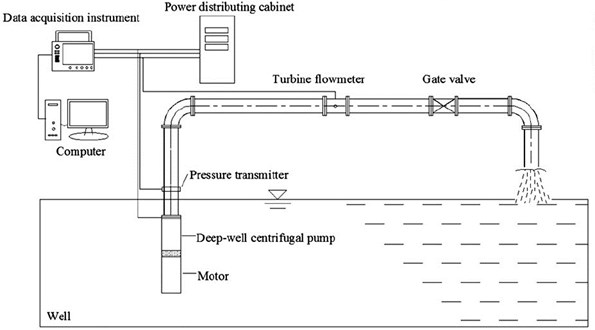### 3.2.6. Experimental results

Fig. 5 is comparison between experimental value and calculating value of multistage pump with the blade thickness of 1.5 mm. It can be seen from Fig. 6, calculating value and efficiency value coincides perfectly and deviation is less than 1 % at design flow rate while calculating flow field is not same as the real one at un-designed flow rate. Hence, the calculating result has more than 3 % deviation compared with experimental result.

There are three main reasons making deviation. Firstly, at small flow rate, the liquid in the impeller and return diffuser is easy to produce flow separation or vortex. Secondly, in order to set modeling and calculation, the model is simplified to some extent in the process of simulation. Thirdly, due to the limitations of stamping process, model of simulation has some deviation to real model. Overall, calculation value is close to experimental value, and numerical calculation is credible at a certain extent. Thus, in order to analyze inner flow field and strength of impeller, numerical simulation in the study is adopted.

Fig. 5Comparison of calculating and experimental value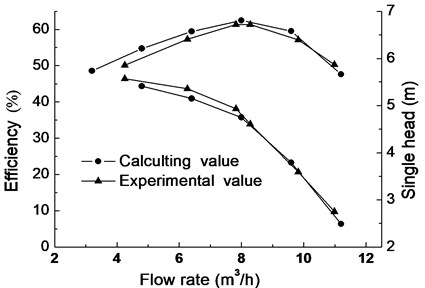### 3.3. Element model of impeller

The finite element analysis of the impeller in this paper is based on ANSYS Workbench and the impeller is made of stainless steel 0Cr18Ni9, whose basic property is listed in Table 7.

Table 7Material properties

 Properties Value Elasticity modulus $E$ (MPa) 193000 Density $\rho$ (kg/m3) 7930 Yielding strength ${\sigma }_{s}$ (MPa) 207 Poisson ratio $\mu$ 0.37

The structural cell of impeller is divided freely, and calculating model has 129654 cells and 248258 nodes. The impeller is connected with quincunx shaft and shaft sleeve is used to realize axial locating between impellers. To set constraint condition conveniently, the intersection between impeller and shaft is simplified as circular holes plane and constrained with cylindrical plane. Loads on impeller include inertial force and surface force. Inertial force is mainly caused by the rotation speed while surface force by the fluid pressure impacting on impeller surface. The interfaces between the impeller and fluid (all of blade surfaces, inner surfaces and outer surfaces of impeller cover) are set as Fluid Solid Interface.

## 4. Calculation results

### 4.1. Effect of blade thickness on pump performance

The theoretical head is drawn from Euler equation of pump:

4
${H}_{t}=\frac{{u}_{2}{v}_{u2}-{u}_{1}{v}_{u1}}{g}=\frac{{u}_{2}}{g}\left({u}_{2}{h}_{0}-\frac{{Q}_{t}}{\pi {D}_{2}{b}_{2}{\varphi }_{2}tg{\beta }_{2}^{}}\right)-\frac{{u}_{1}{v}_{u1}}{g},$
5
${\varphi }_{2}=1-\frac{z{s}_{u2}}{\pi {D}_{2}}=1-\frac{z\delta }{\pi {D}_{2}\mathrm{s}\mathrm{i}\mathrm{n}{\beta }_{2}\mathrm{s}\mathrm{i}\mathrm{n}\gamma },$
6
${h}_{0}=1-\left(\frac{\pi }{z}\right)\mathrm{s}\mathrm{i}\mathrm{n}{\beta }_{2}.$

In the equations above, ${u}_{1}$ and ${u}_{2}$ are peripheral speed at blade inlet and outlet, ${v}_{u1}$ and ${v}_{u2}$ are peripheral component of absolute speed at inlet and outlet, $g$ is the acceleration of gravity, ${Q}_{t}$ refers to theoretical flow, ${h}_{0}$ is Stodala slip coefficient, ${D}_{2}$ is outlet diameter of impeller, ${\beta }_{2}$ is blade outlet angle, $z$ is number of blades, and ${\phi }_{2}$ is outlet crowding coefficient. Law could be found from Eqs. (4) and (5) that smaller ${\phi }_{2}$, that is, thicker blades may bring steeper head curve. Under the same conditions, five impeller models with different blade thicknesses are calculated by ANSYS Fluent, and the performance curves are shown in Fig. 6.

Fig. 6Performance curve with different blade thicknesses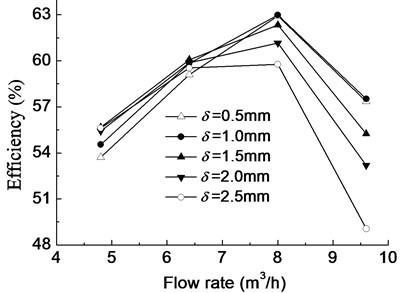a) Efficiency curve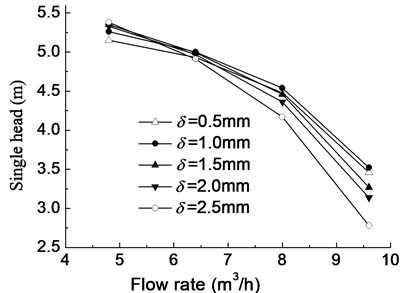According to Fig. 3, the inlet section and outlet section are at the same height, so ${z}_{d}-{z}_{s}=$0, and the Eq. (1) can be simplified to the Eq. (7) as follows:

7
$H=\frac{{p}_{d}-{p}_{s}}{\rho g}+\frac{{v}_{d}^{2}-{v}_{s}^{2}}{2g}=\frac{{P}_{d}}{\rho g}-\frac{{P}_{s}}{\rho g}.$

In the Eq. (7), ${P}_{d}$ and ${P}_{s}$ show the total pressure on outlet and inlet section, and theycan be obtained through the post-processing in the ANSYS Fluent, so the head $H$ can be calculated through the Eq. (7). Moreover, the sum torque on shaft $M$ can also be gained through ANSYS Fluent, the flow rate $Q$ and the angular velocity of impeller are known quantities, so the efficiency $\eta$ can be calculated through the Eq. (2).

Table 8Stress distribution for impellers with different thicknesses

 Blade thickness Inertia load Fluid load Combination of both loads 0.5 mm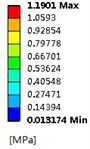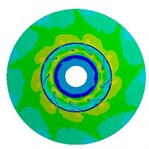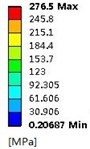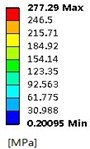1.5 mm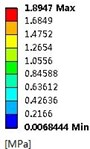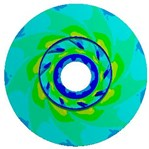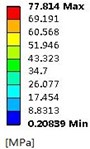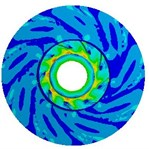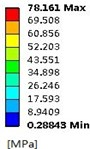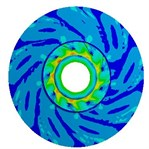2.5 mm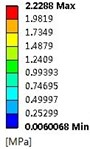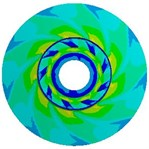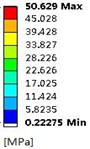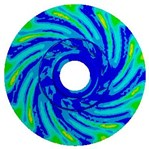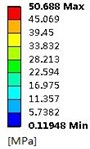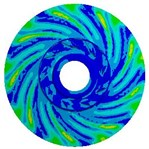### 4.2. Effects of impeller loads on stress

Loads on stainless steel impeller consist of inertial force load caused by impeller rotation speed and fluid pressure load when pump is working. In order to compare the influence of the two loads on impeller, this study calculates respectively inertial force load, fluid pressure load, and both loads at design flow rate on stress and deformation of impeller. Table 8 means the stress distribution of impellers whose blade thicknesses are 0.5 mm, 1.5 mm, and 2.5 mm. The table indicates stress distributions on three impellers are similar. The maximum stress by fluid pressure load is much greater than those brought by inertial force load, while slightly smaller than those brought by both of them, which means that the load brought by high-pressure fluid plays a dominating role in stress distribution. Affected by three different loads, stress on impeller distributes in circumference and it is apparently larger at the inlet of blade. Besides, when the radius is the same, the stress at intersection between impeller and cover is obviously larger. With the increase of blade thickness, the maximum stress caused by inertial force load gradually increases, while the maximum stress of fluid pressure load decreases. That’s because thicker blade brings heavier impeller and larger stress caused by inertial force. Moreover, thicker blade increases the area of intersection between blade and cover, which makes impeller steady and reduces the maximum stress caused by fluid pressure. If the blade thickness is 0.5 mm, the maximum stress caused by both of the two loads is 277.29 MPa (kg/cm2) which exceeds the stainless steel’s yield strength ${\sigma }_{s}=$207 MPa; if it is 1.5 mm and 2.5 mm, the maximum stress are respectively 78.16 MPa (kg/cm2) and 50.69 MPa (kg/cm2). But the over-thick blade not only increases the cost of impeller, but also reduces the performance of pump. Hence, as a reasonable value of blade thickness of impeller, 1.5 mm is selected at last.

Table 9Deformation distribution for impellers with different thicknesses

 Blade thickness Inertia load Fluid load Combination of both loads 0.5 mm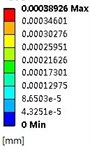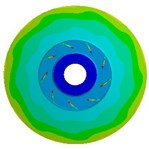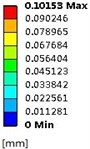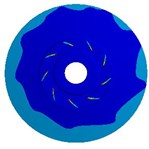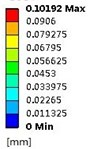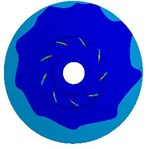1.5 mm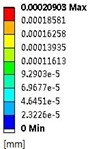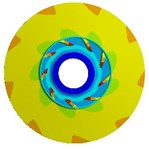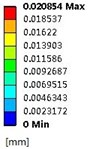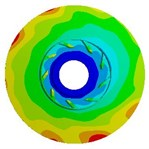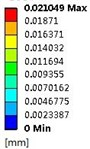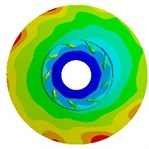2.5 mm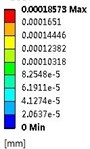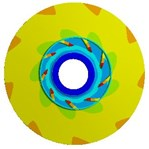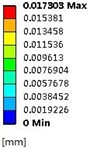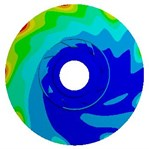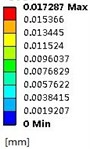## 5. Conclusions

1) At design flow rate and large flow rate, the efficiency and single-stage head of stainless steel multistage centrifugal pump decrease as the increase of impeller blade thickness while instead at small flow rate, which indicates that the best efficiency point of the pump shifts to the small flow direction with the increase of impeller blade thickness.

2) Through the finite element calculation of the impeller with different blade thicknesses, distribution patterns of stress and deformation caused by inertial force load and fluid pressure load are found.

3) When the blade thickness is from 0.5 mm to 1.5 mm, the efficiency and head change a little at design flow rate; if it is more than 1.5 mm, the efficiency and head decrease rapidly with the increase of thickness. With comprehensive consideration of pump performance and strength, the blade thickness of 1.5 mm is selected at last.

15 February 2014
Accepted
26 May 2014
Published
30 June 2014
Keywords
energy-saving
stainless steel impeller
multistage pump
finite element
fluid solid interaction
Acknowledgements

This research was supported by the Priority Academic Program Development of Jiangsu Higher Education Institutions, the National Natural Science Foundation of China (Grant No. 51279069), the National Natural Science Foundation of Jiangsu Province (Grant No. BK20131256), the Graduate Innovation Project of Jiangsu Province (CXLX12_0642), the National Science and Technology Support Project (2011BAF14B01), Science and Technology Support Project of Jiangsu Province (BE2012150).